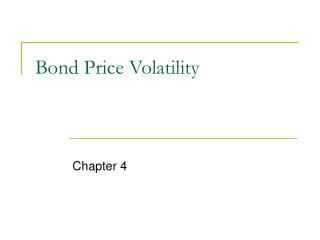Download PresentationBond Price Volatility

# Bond Price Volatility - PowerPoint PPT Presentation

Bond Price Volatility. Chapter 4. Price Volatility Characteristics. Price Volatility of Option-Free Bond. Although the prices of all option-free bonds move in opposite direction from the change in yield required, the % price change is not the same for all bonds.I am the owner, or an agent authorized to act on behalf of the owner, of the copyrighted work described.
Download Presentation## Bond Price Volatility

An Image/Link below is provided (as is) to download presentation

Download Policy: Content on the Website is provided to you AS IS for your information and personal use and may not be sold / licensed / shared on other websites without getting consent from its author.While downloading, if for some reason you are not able to download a presentation, the publisher may have deleted the file from their server.

- - - - - - - - - - - - - - - - - - - - - - - - - - E N D - - - - - - - - - - - - - - - - - - - - - - - - - -
Presentation Transcript
1. Bond Price Volatility Chapter 4

2. Price Volatility Characteristics

3. Price Volatility of Option-Free Bond • Although the prices of all option-free bonds move in opposite direction from the change in yield required, the % price change is not the same for all bonds. • For very small changes in the yield required, the % price change for a given bond is roughly the same, whether the yield required increases or decreases. • For large changes in the required yield, the % price change is not the same for an increase in the required yield as it is for a decrease in the required yield. • For a given large change in basis points, the % price increase is greater than the % price decrease.

4. Price Volatility • For a given term to maturity and initial yield, the price volatility of a bond is greater, the lower the coupon rate. • For a given coupon rate and initial yield, the longer the term to maturity, the greater the price volatility. • The higher the YTM at which a bond trades, the lower the price volatility.

5. Measures of Price Volatility • price value of a basis point – gives dollar price volatility not % • yield value of a price change • duration

6. Duration

7. Duration

8. Duration

9. Modified Duration

10. Duration • duration is less than (coupon bond) or equal to (zero coupon bond) the term to maturity • all else equal, • the lower the coupon, the larger the duration • the longer the maturity, the larger the duration • the lower the yield, the larger the duration • the longer the duration, the greater the price volatility

11. Duration • dollar duration = (-MD) * P • spread duration – measure of how a non-Treasury bond’s price will change if the spread sought by the market changes • spread duration = 0 for Treasury • for fixed rate security it is the approximate change in the price of a fixed-rate bond for a 100 bp change in the spread • for a floater, a spread duration of 1.4 means that if the spread the market requires changes by 100 bp, the floater’s price will change by about 1.4% • portfolio duration – weighted average of bonds’ durations

12. Portfolio Duration

13. Portfolio Duration

14. Measures of Bond Price Volatility

15. Price-Yield Relationship

16. Price Approximation using Duration

17. Convexity • second derivative of price-yield is dollar convexity measure of bond • convexity measure • convexity measure in terms of periods squared so to convert to annual figure, divide by 4

18. Convexity consider the 25-year 6% bond selling at 70.357 to yield 9%

19. % Price Change • consider a 25 year 6% bond selling to yield 9% • MD = 10.62, convexity = 182.92 • required yield increases 200 bp from 9% to 11% • estimated price change due to duration and convexity is -21.24% + 3.66% = -17.58%

20. implication of convexity for bonds when yields change market takes convexity into account when pricing bonds but to what extent should there be difference? Convexity

21. As the required yield increases (decreases), the convexity of a bond decreases (increases). This property is referred to as positive convexity. For a given yield and maturity, the lower the coupon, the greater the convexity of a bond. For a given yield and modified duration, the lower the coupon, the smaller the convexity. Convexity

22. Approximating Duration • Use the 25 year, 6% bond trading at 9%. Increase the yield by 10bp from 9% to 9.1%. So ∆y = 0.001. The new price is P+ = 69.6164. • Decrease the yield on the bond by 10 bp from 9% to 8.9%. The new price is P- = 71.1105. • Because the initial price, P0, is 70.3570, the duration can be approximated as follows

23. Approximating Duration • Increase the yield on the bond by a small number of bp and determine the new price at this higher yield level. New price is P+. • Decrease the yield on the bond by the same number of bp and calculate the new price. P- • Letting P0 be the initial price, duration can be approximated using the following where ∆y is the change in yield used to calculate the new prices. This gives the average % price change relative to the initial price per 1-bp change in yield.

24. Approximating Convexity